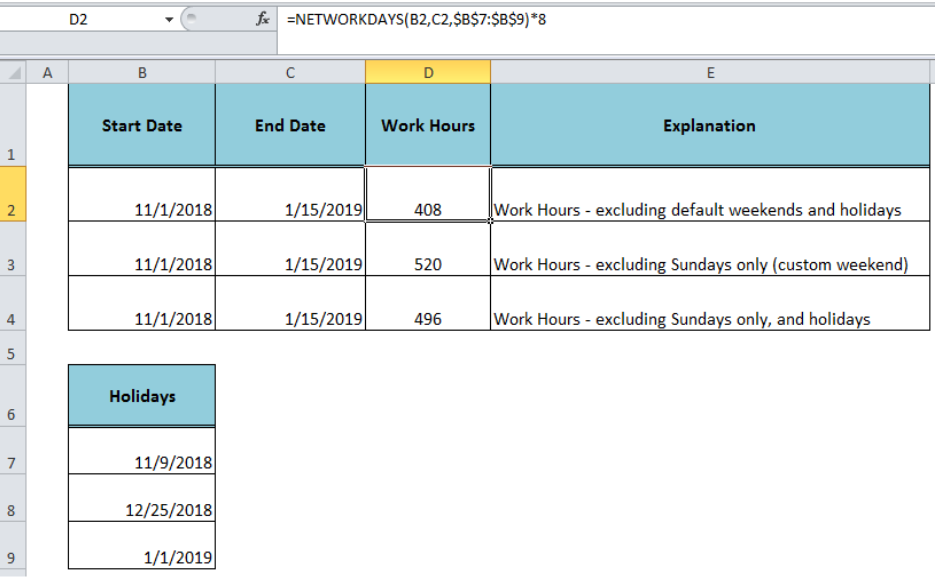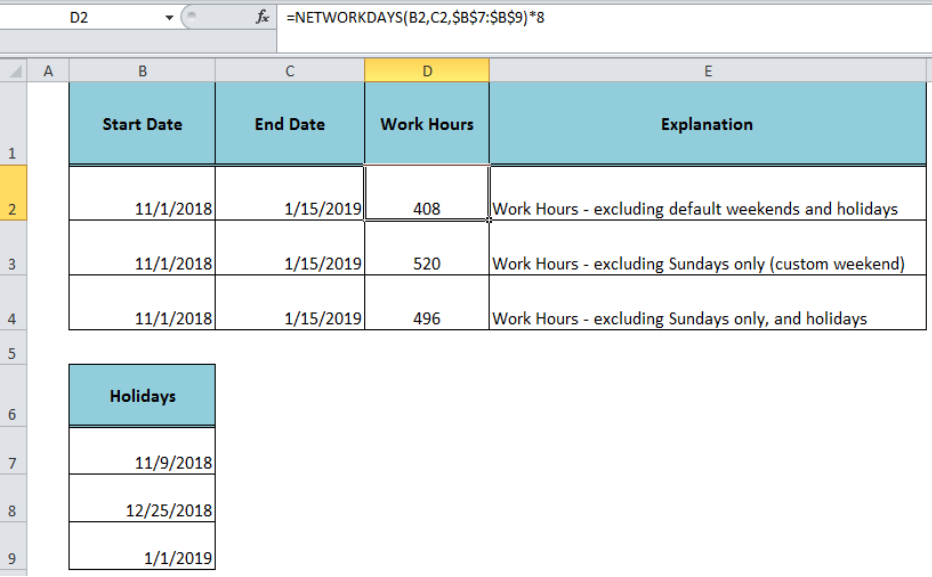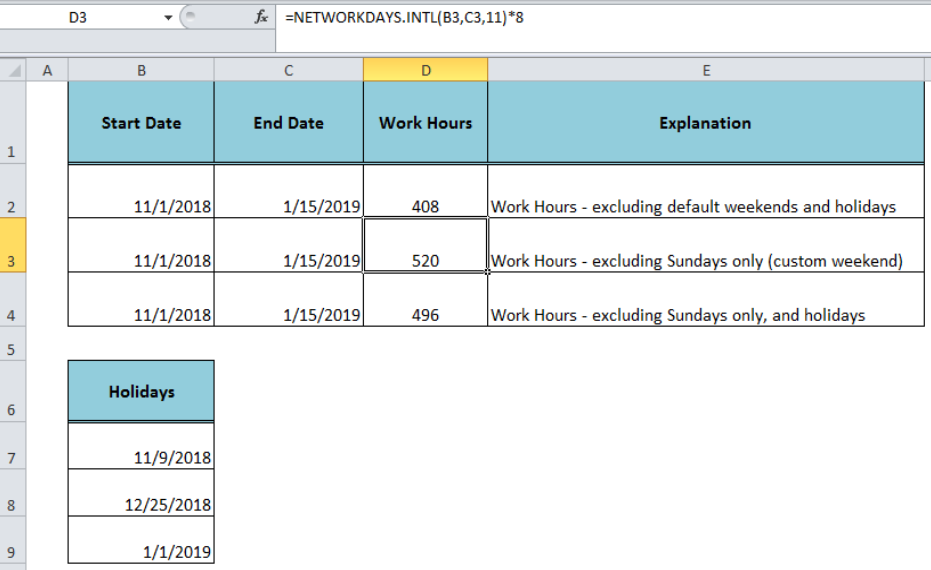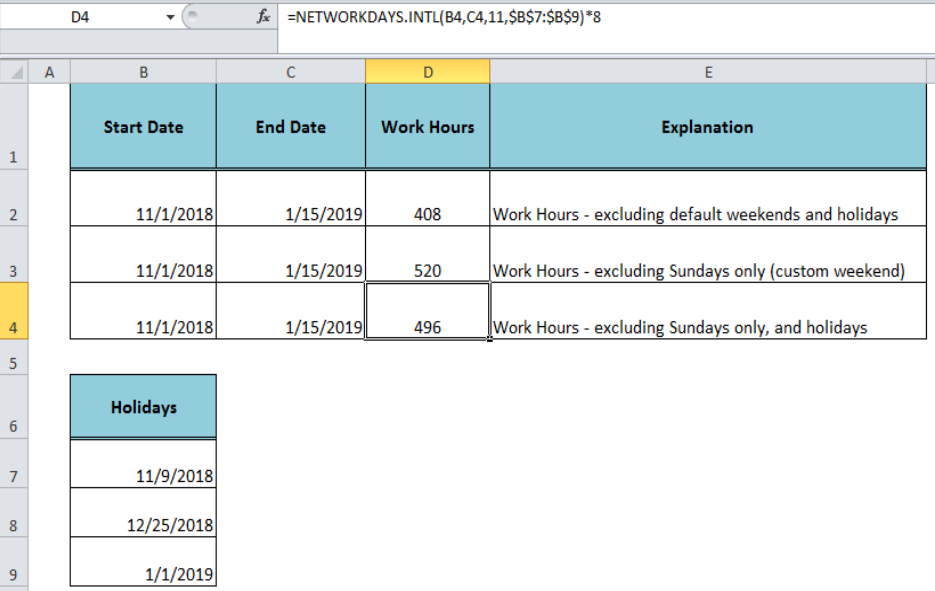Get instant live expert help with Excel or Google Sheets“My Excelchat expert helped me in less than 20 minutes, saving me what would have been 5 hours of work!”

#### Post your problem and you’ll get expert help in seconds.

Your message must be at least 40 characters
Our professional experts are available now. Your privacy is guaranteed.

# How to Get Work Hours Between Dates in ExcelRead time: 34 minutes

We may need to calculate work hours between two dates when workers are paid for working hours between dates. We can achieve this by calculating work days between two dates excluding the weekends and holidays between two dates and then multiplying it with a specified number of working hours in a workdayFigure 1. Calculating Work Hours Between Dates

## Work Hours – Excluding Default Weekends and Holidays

To calculate work hours between dates excluding default weekends (Saturday and Sunday) and holidays we need to use a formula based on the Excel NETWORKDAYS function as per the following generic syntax;

`=NETWORKDAYS(start_date, end_date, [holidays]) * daily_working_hours`

The Excel NETWORKDAYS function returns net workdays between two dates and we will multiply the net workdays by the number of working hours in a workday to get the result. Suppose we have the start date in cell B2 and end date in cell C2 and holidays in range B7: B9, and the number of working hours per day are 8. We need to use the following formula in cell D2;

`=NETWORKDAYS(B2, C2,\$B\$7:\$B\$9)*8`Figure 2. Work Hours Between Dates – Excluding Default Weekends and Holidays

## Work Hours – Excluding Custom Weekends Only

If we want to calculate the work hours between two dates excluding only custom weekends, say Sunday, without taking holidays into account, then we need to use the Excel NETWORKDAYS.INTL function as per following formula syntax;

`=NETWORKDAYS.INTL(start_date, end_date, [weekend]) * daily_working_hours`

The Excel NETWORKDAYS.INTL function returns the number of workdays between two dates with custom weekend parameters. As we want to exclude custom weekend Sunday only, by ignoring the holidays, so we need to use the following formula in cell D3;

`=NETWORKDAYS.INTL(B3,C3,11)*8`Figure 3. Work Hours Between Dates – Excluding Custom Weekends Only

## Work Hours – Excluding Custom Weekends and Holidays

We will use the following formula syntax to get the work hours between two dates excluding the custom weekends and holidays;

`=NETWORKDAYS.INTL(start_date, end_date, [weekend], [holidays]) * daily_working_hours`

In our example, we use the following formula in cell D4 to calculate the work hours excluding Sundays (custom weekend) and holidays;

`=NETWORKDAYS.INTL(B4,C4,11,\$B\$7:\$B\$9)*8`Figure 4. Work Hours Between Dates – Excluding Custom Weekends and Holidays

## Instant Connection to an Expert through our Excelchat Service:

Most of the time, the problem you will need to solve will be more complex than a simple application of a formula or function. If you want to save hours of research and frustration, try our live Excelchat service! Our Excel Experts are available 24/7 to answer any Excel question you may have. We guarantee a connection within 30 seconds and a customized solution within 20 minutes.

### Did this post not answer your question? Get a solution from connecting with the expert.Another blog reader asked this question today on Excelchat:
Solution examplesi have this formula currently which works but not if I want it for every day - any ideas ?? (NETWORKDAYS.INTL(L1362,M1362,11,BH!A:A)-1)*("17:30"-"8:30")+IF(NETWORKDAYS.INTL(M1362,M1362,11,BH!A:A),MEDIAN(MOD(M1362,1),"8:30","17:30"),"17:30")-MEDIAN(NETWORKDAYS.INTL(L1362,L1362,11,BH!A:A)*MOD(L1362,1),"8:30","17:30")
Solved by A. D. in 28 minsneed help with the different sheets and formula IF(A4=B4,0,NETWORKDAYS(A4+1,B4,\$E\$9:\$E\$42) +0.5*(INT((+B4-A4+1)/7)+IF(WEEKDAY(A4+1)+MOD (B4-A4-1,7)>=7,1,0)))
Solved by O. D. in 11 mins## Subscribe to Excelchat.coAnother blog reader asked this question today on Excelchat: